# S&P500 Daily stock Returns

D. Spinellis, AUEB

## Project Description

The project is based on the paper Regularized Robust Portfolio Estimation by T. Evgeniou, M. Pontil, D. Spinellis, R. Swiderski, and N. Nassuphis.

It describes a simple analysis of daily stock returns of S&P 500 stocks.

Disclaimer:

This project is meant to be an example of how to organize a data analytics case study/project. It is not meant to provide insights for stock data or stock trading. It also does not build on any finance literature (e.g. regarding risk factors such as size, growth, or momentum).

The returns generated may also be different from the returns of, say, the S&P 500 index, as the universe of stocks/data used may be biased (e.g. survivorship bias).


## The Data

10 years (from 2003-01-03 to 2013-04-12) of daily returns of 423 companies which were in the S&P500 index in February 2013. Every row is a day and every column is an individual stock. The data matrix has 2586 rows and 423 columns.

This is the histogram of the daily stock returns across all these stocks during this time period: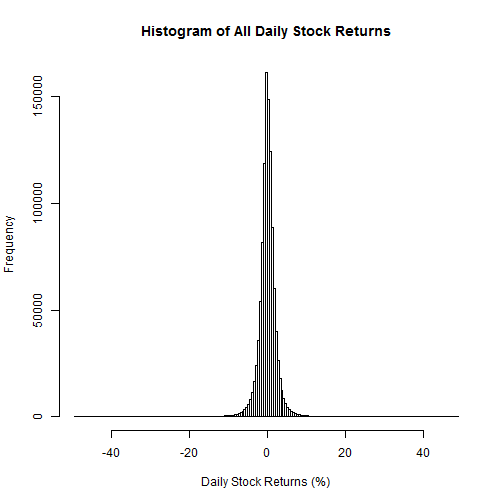The equal-weight average of these stocks (the “equal weight market”) has performed as follows: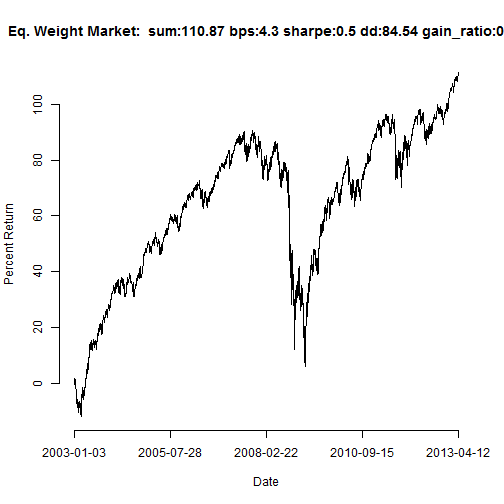where dd is the maximum drawdown and gain_ratio is the percentage of the days the market had positive returns.

All returns reported correspond to the total sum of returns if we invest every day 1 dollar. For example, in this case the market returns is 110.8691%, which means that we would have made a total of 110.8691% of 1 dollar, namely 1.1087 dollars. If the return was, say, -200%, we would have lost 2 dollars.

Here are the monthly and yearly returns of this market:

Jan Feb Mar Apr May Jun Jul Aug Sep Oct Nov Dec Year
2003 -5.80 -1.40 1.30 8.00 8.90 1.20 2.70 4.50 -1.60 7.10 2.10 4.20 31.20
2004 1.60 2.80 0.30 -2.10 2.20 3.20 -3.60 -0.20 3.60 2.10 5.90 3.60 19.60
2005 -2.20 3.20 -1.10 -3.20 4.70 1.80 5.70 -0.90 1.10 -2.00 4.30 0.70 12.20
2006 4.90 -0.10 2.30 1.00 -3.40 0.30 -1.60 2.60 2.10 3.50 2.80 0.20 14.70
2007 2.70 -0.40 1.10 4.10 3.40 -1.90 -3.30 1.10 3.20 2.20 -4.30 -0.90 7.10
2008 -5.50 -1.90 -0.60 5.40 3.40 -9.50 -0.70 2.40 -10.10 -23.50 -10.60 3.10 -48.30
2009 -8.20 -11.80 9.20 14.40 4.50 -0.20 9.00 4.20 4.80 -3.00 5.20 4.30 32.30
2010 -3.80 4.00 6.40 3.00 -8.00 -6.30 6.60 -5.10 9.70 3.40 1.00 6.90 18.00
2011 2.00 3.90 0.90 2.80 -0.70 -1.90 -3.80 -6.60 -9.30 12.40 -0.30 0.00 -0.80
2012 5.20 3.70 2.30 -0.90 -7.50 3.40 0.30 2.60 2.00 -1.00 0.90 2.10 13.20
2013 5.90 1.10 3.80 0.90 0.00 0.00 0.00 0.00 0.00 0.00 0.00 0.00 11.70

Summary Statistics Daily Market Returns
V1 V2 V3 V4 V5 V6
Min. :-10.543 1st Qu.: -0.514 Median : 0.099 Mean : 0.043 3rd Qu.: 0.673 Max. : 10.948

And this is an Interactive chart: (Put the mouse on the plot to see daily values, and zoom using click-and-drag with the mouse in the smaller graph below)

If we select with hindsight the best individual stock in terms of returns, it performs as follows: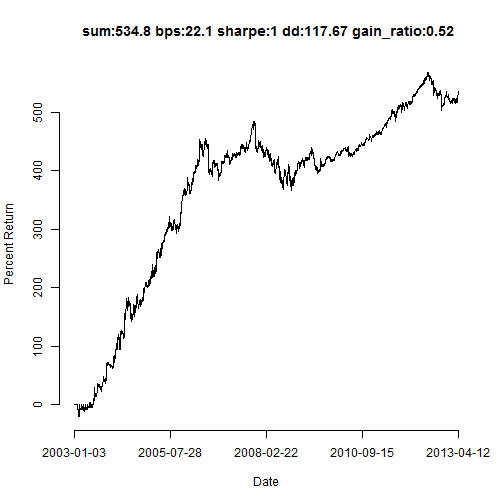while the worst one is: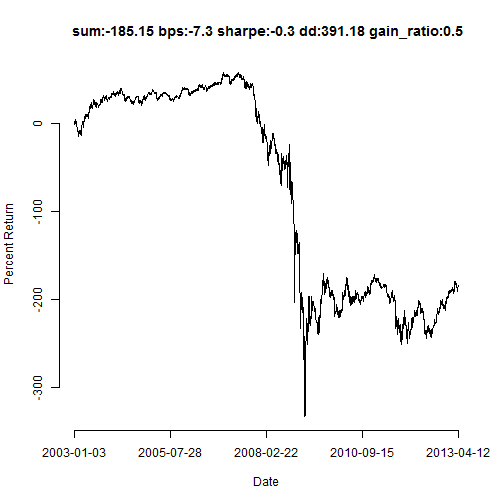These company tickers are MNST and C, respectively. If we were to select them using their Sharpe, the best and worst stocks would have been AAPL and C, respectively.

## An Analysis

We will build on the basic mean-reverting strategy from The Econometrics of Financial Markets by J. Campbell, A. Lo, and C. MacKinlay.

mr_strategy = matrix(-sign(shift(market, 1)) * market, ncol = 1)
colnames(mr_strategy) <- "Market Mean Reversion"
rownames(mr_strategy) <- rownames(market)


which, when applied to the equally weighted market performs as follows: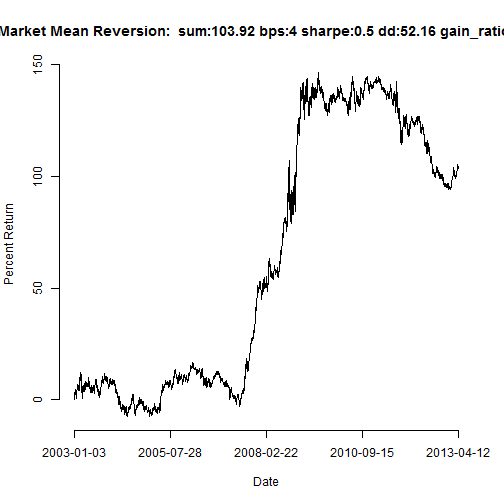We see the special period during the financial crisis.

Here are the monthly and yearly returns of this mean reversion strategy:

Jan Feb Mar Apr May Jun Jul Aug Sep Oct Nov Dec Year
2003 6.10 3.20 -4.30 0.90 2.00 -3.10 3.40 -5.90 6.00 0.90 -2.70 0.30 7.00
2004 0.40 -5.00 -5.40 -1.40 -2.20 2.80 5.90 -4.70 2.70 -2.20 -2.30 0.60 -10.80
2005 -1.70 1.80 -0.10 6.70 3.00 1.70 0.20 2.40 -1.10 0.10 -0.50 0.50 13.00
2006 2.10 4.40 -1.30 -2.20 1.30 -6.40 0.70 -0.90 3.00 2.70 -4.90 -0.80 -2.20
2007 0.20 -4.90 -0.40 -2.40 0.40 2.80 8.30 5.90 8.90 6.80 16.20 1.00 43.00
2008 4.90 -6.20 11.70 -6.70 3.10 2.90 14.60 5.90 25.90 -23.10 1.40 39.40 73.80
2009 11.20 -2.60 7.70 -4.60 2.70 -1.20 2.80 -2.40 -4.50 1.30 2.70 -2.40 10.60
2010 2.80 1.50 -4.90 -4.70 9.20 -3.50 0.00 2.00 1.40 5.50 -3.40 -0.20 5.60
2011 4.40 -2.40 -2.70 -1.80 -2.60 0.80 -1.30 -6.30 -7.70 4.80 -6.40 5.50 -15.70
2012 -1.20 2.40 -1.00 -3.40 -6.40 -4.40 -7.70 -0.90 0.00 -2.00 -3.90 0.90 -27.50
2013 -1.60 7.30 -0.70 2.20 0.00 0.00 0.00 0.00 0.00 0.00 0.00 0.00 7.20

If we were to implement this only the days when the previous day the market fell, this would perform as follows: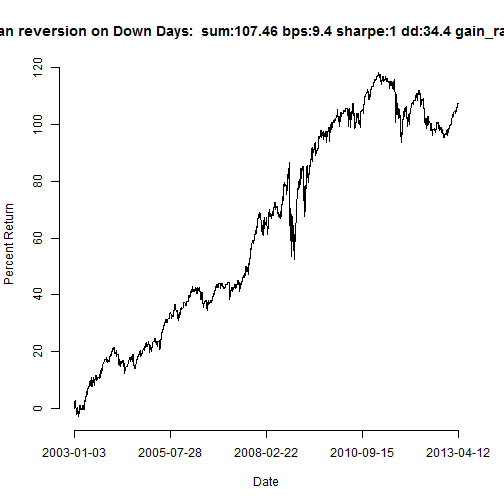while the days when the previous day the market rose, this performed as follows: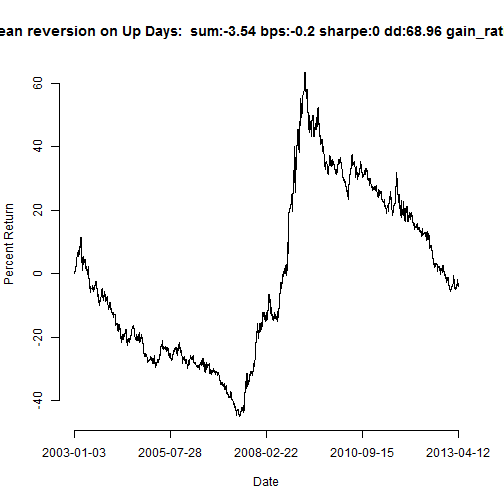with montly returns as follows:

Here are the monthly and yearly returns of this “down market days only”“ mean reversion strategy:

Jan Feb Mar Apr May Jun Jul Aug Sep Oct Nov Dec Year
2003 0.30 0.90 -1.50 4.40 5.50 -0.90 3.10 -0.70 2.20 4.00 -0.30 2.30 19.20
2004 1.00 -1.10 -2.60 -1.70 0.00 3.00 1.20 -2.50 3.20 0.00 1.80 2.10 4.40
2005 -1.90 2.50 -0.60 1.80 3.90 1.80 3.00 0.70 0.00 -1.00 1.90 0.60 12.60
2006 3.50 2.10 0.50 -0.60 -1.00 -3.10 -0.40 0.80 2.60 3.10 -1.10 -0.30 6.20
2007 1.50 -2.60 0.40 0.80 1.90 0.50 2.50 3.50 6.00 4.50 6.00 0.10 25.10
2008 -0.30 -4.10 5.60 -0.60 3.20 -3.30 7.00 4.10 7.90 -23.30 -4.60 21.20 12.70
2009 1.50 -7.20 8.40 4.90 3.60 -0.70 5.90 0.90 0.10 -0.90 4.00 1.00 21.50
2010 -0.50 2.80 0.80 -0.80 0.60 -4.90 3.30 -1.50 5.60 4.40 -1.20 3.30 11.80
2011 3.20 0.70 -0.90 0.50 -1.70 -0.60 -2.60 -6.40 -8.50 8.60 -3.40 2.70 -8.20
2012 2.00 3.10 0.60 -2.20 -7.00 -0.50 -3.70 0.90 1.00 -1.50 -1.50 1.50 -7.20
2013 2.20 4.20 1.50 1.50 0.00 0.00 0.00 0.00 0.00 0.00 0.00 0.00 9.50

The difference in bevavior is quite visible.

Moreover, we can clearly see the financial crisis (and probably that there are different market regimes).

If we select with hindsight the best individual stock in terms of returns for this simple strategy (the most mean reverting S&P500 stock the past 10 years), it performs as follows: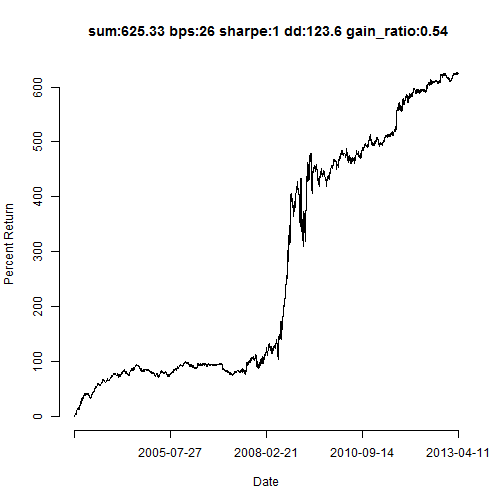while the worst one (the least mean reverting S&P500 stock the past 10 years) is: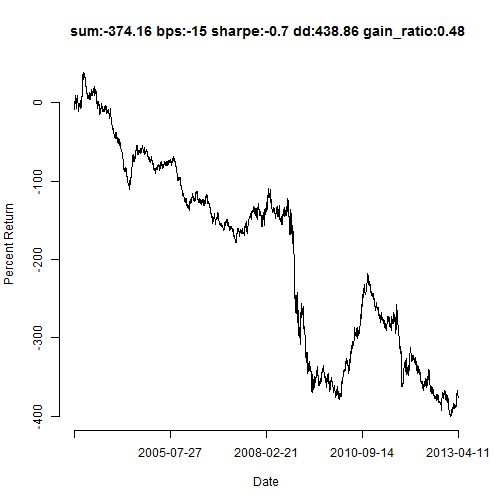These company tickers are HBAN and MU, respectively. If we were to select them using their Sharpe, the best and worst stocks would have been PCL and F, respectively.

The "market” of the mean-reverting strategies is: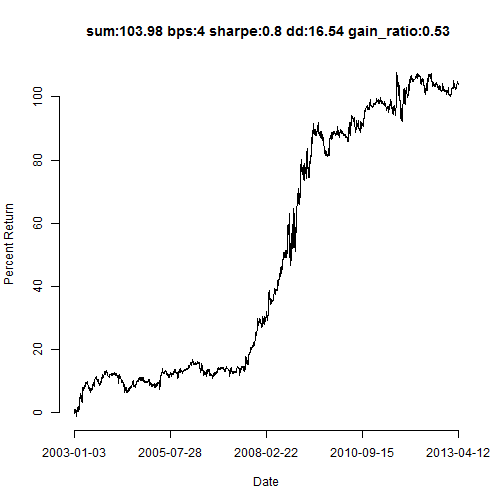Notice that one could also use the following momentum strategy instead:

mom_strategy = sign(shift(market, 1)) * market
names(mom_strategy) <- rownames(market)


which would lead to the exact opposite returns when used for the market. Clearly MU has now the best returns based on this momentum strategy.

If we could separate the stocks into momentum and mean reverting (e.g. for each stock select the one of the two that leads to better returns or Sharpe), the average of those series would be: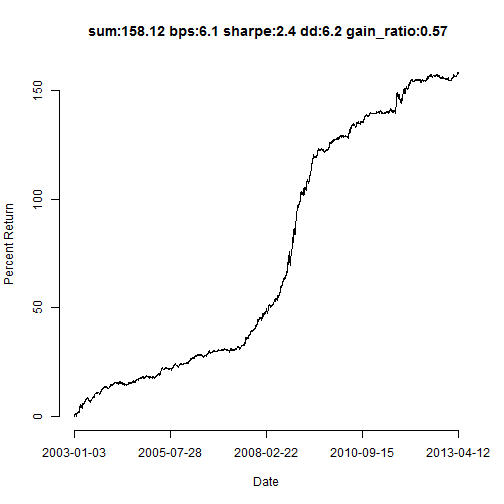Of course one could do this selection for shorter time windows to achieve even better returns. For example, these are the returns of the recent third of the days, namely the last 862 days: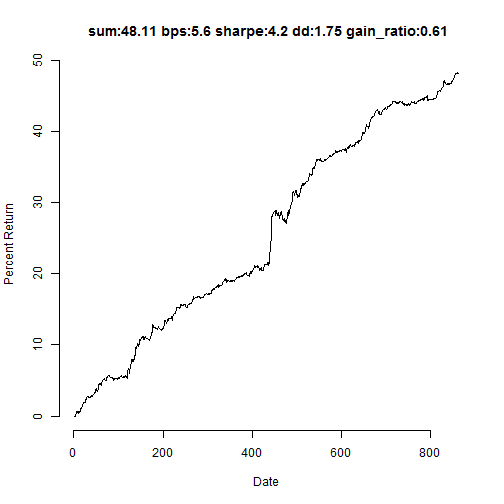The returns and Sharpe look great, but making this selection between momentum and mean-reversion for each stock without hindsight is of course not practical.

## Risk Factors

Instead of applying these simple mean-reverting and momentum strategies to the actual daily stock returns, one can do so on residuals of the stock returns after regressing individual stocks on (what one could call) risk factors.

Note: For computational reasons and simplicity, all the analysis in this note is performed with hindsight. One could perform the exact same analysis using a rolling window (e.g. of 250 or 60 days for example), doing every day the same analysis using the data in the corresponding window and deciding the stocks to trade the next day.

We will first perform a simple Principal Component Analysis of our data. This will cleate the portfolios with the largest variance. We will then regress each stock on the principal components (using for example linear regression) and estimate the residuals of these regressions. We can then use the exact same mean-reverting and momentum strategies above, but this time for the residuals (which are returns of long-short portfolios, corresponding to the estimated regressions).

Let's first see how many eigenvalues we need to capture a reasonable percentage of the variance in our data. The eigenvalues of this data lead to the following scree plot: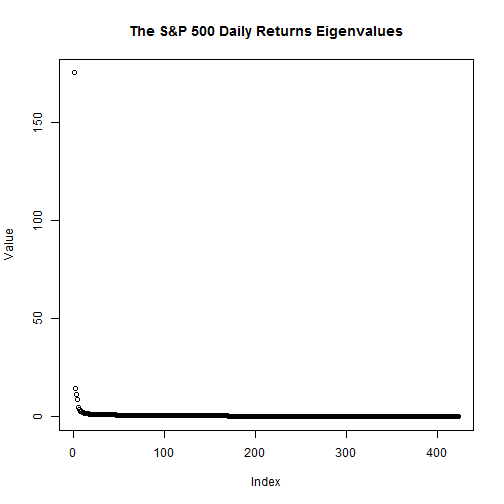There is one very large eigenvalue: how would the corresponding largest eigen-portfolio look like?

As we can also see from the table below, the top 5 eigenvectors capture 50% of the variance in the S&P 500 daily stock data:

Eigenvalues and Variance Explained
eigenvalue percentage of variance cumulative percentage of variance
comp 1 175.220 41.423 41.423
comp 2 14.593 3.450 44.873
comp 3 11.487 2.716 47.589
comp 4 8.789 2.078 49.666
comp 5 4.944 1.169 50.835

Let's now see the first principal component of the data. We can plot the returns of the largest PCA component of the S&P 500 data as follows:

SP500PCA_simple = eigen(cor(ProjectData))
PCA_first_component = ProjectData %*% norm1(SP500PCA_simple$vectors[, 1]) if (sum(PCA_first_component) < 0) { PCA_first_component = -PCA_first_component flipped_sign = -1 } else { flipped_sign = 1 } names(PCA_first_component) <- rownames(market) pnl_plot(PCA_first_component)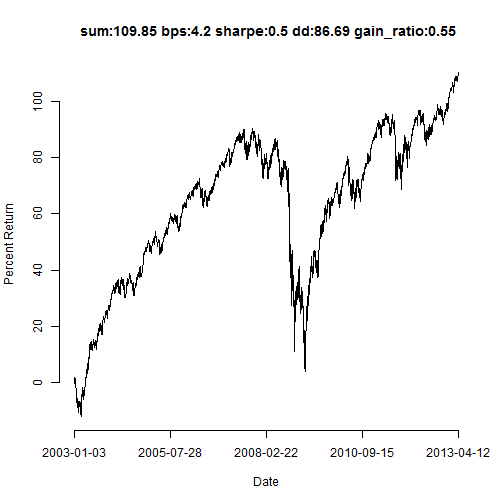Do you see the similarity with the returns of the market above? The correlation between the equal weighted market and the first principal component portfolio is 0.9998. ** The first principal component, explaining 1.7522 × 104% of the variance in the data, is the market, as expected. Indeed, the weights of the first principal component on the individual stocks are: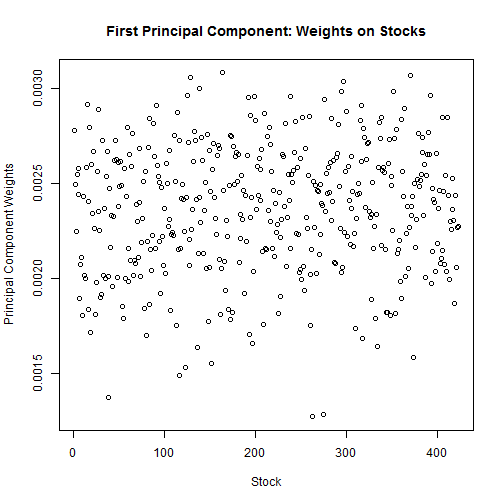As we see, almost all stocks have the same positive weight 1/423=0.0024. How about the second component? This is how this one performs: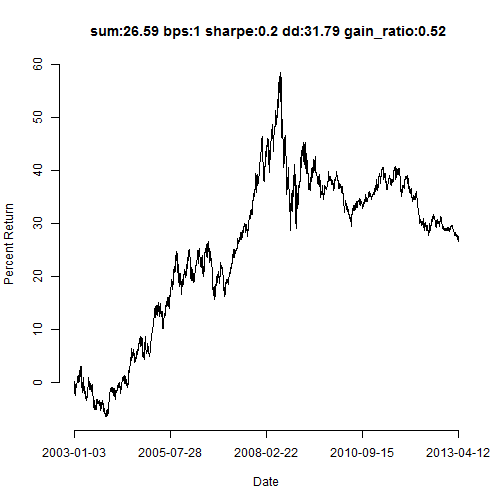The weights of this component on the stocks are: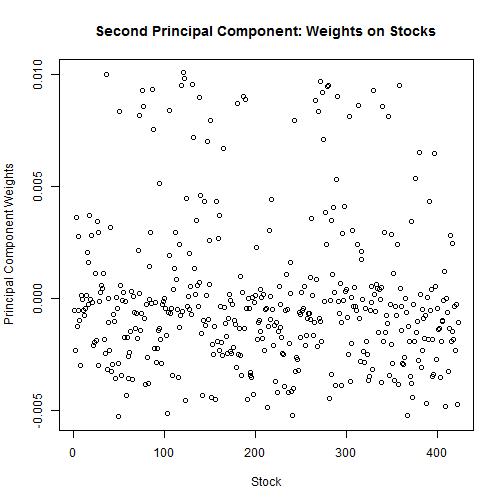Notice that these are both positive and negative. We can also use a rotation to make the components sparser. These are the top 10 stocks with the largest positive weight: DVN, APA, DO, NOV, EOG, DNR, SWN, NBL, NE, CHK, while these are the top 10 stocks with the largest negative weights: BBT, STI, MTB, CMA, JPM, WFC, ZION, USB, DLTR, FHN. Most of the companies for the second principal component for this time period are from the financial and the energy sectors. Let's now use the first 3 principal components as our “risk factors” and estimate the linear regression residuals of all our stocks using these compoments as independent variables. Here is the code tha replaces the original daily returns with the residuals of the stocks when regressed on these factors: Although formally we need to de-mean the data in the calculations below, and also use a regression constant (“alpha”), one could still ignore these mathematical formalisms and set these means and alpha to 0 - since in practice going forward one cannot assume these would remain constant or have any value different from 0. Afterall if we know the market (mean) returns in the future we would not need any of these analysis. Hence we assume all means and alphas are 0. SP500PCA_simple <- eigen(cor(ProjectData)) TheFactors = SP500PCA_simple$vectors[, 1:numb_components_used]
TheFactors = apply(TheFactors, 2, function(r) if (sum(ProjectData %*% r) < 0) -r else r)
TheFactors = apply(TheFactors, 2, function(r) norm1(r))
Factor_series = ProjectData %*% TheFactors
demean_IVs = apply(Factor_series, 2, function(r) r - use_mean_alpha * mean(r))
ProjectData_demean = apply(ProjectData, 2, function(r) r - use_mean_alpha *
mean(r))
XXtY = (solve(t(demean_IVs) %*% demean_IVs) %*% t(demean_IVs))
stock_betas = XXtY %*% (ProjectData_demean)
Ybar = t(stock_betas) %*% matrix(apply(Factor_series, 2, mean), ncol = 1)
stock_alphas = apply(ProjectData_demean, 2, mean) - Ybar
stock_alphas = use_mean_alpha * matrix(stock_alphas, nrow = 1)
stock_alphas_matrix = rep(1, nrow(ProjectData)) %*% stock_alphas
# make sure each residuals portfolio invests a total of 1 dollar.
stock_betas_stock = apply(rbind(stock_betas, rep(1, ncol(stock_betas))), 2,
norm1)
stock_betas = head(stock_betas_stock, -1)  # last one is the stock weight
stock_weight = rep(1, nrow(ProjectData)) %*% tail(stock_betas_stock, 1)
Stock_Residuals = stock_weight * ProjectData - (Factor_series %*% stock_betas +
stock_alphas_matrix)
rownames(Stock_Residuals) <- rownames(ProjectData)


As before, if we now use the residuals and we select With hindsight the best individual stock (trading its residuals by buying the stock and shorting the risk factor using the estimated regression coefficients, scaled to trade 1 dollar) in terms of returns, it performs as follows: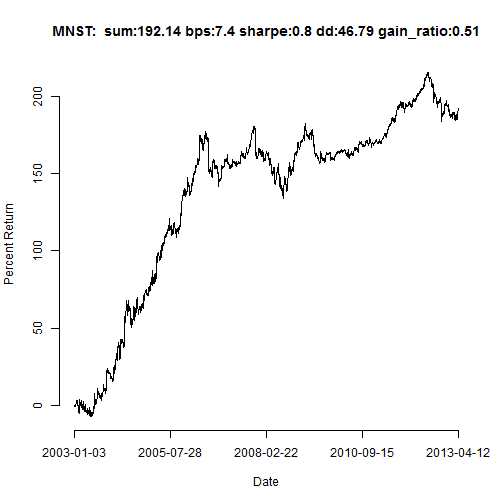while the worst one is: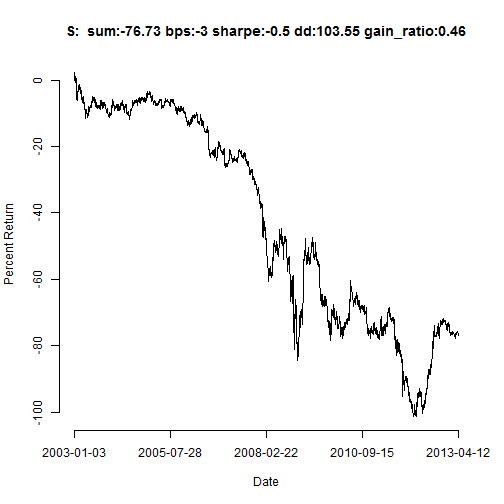These company tickers are MNST and S, respectively.

Note that “trading the residuals” implies that every day we trade the portfolios corresponding to the residuals (with portfolio weights given by the estimated “betas”, scaled to invest 1 dollar every day).

One can now also explore mean reversion or momentum of the residuals. There are the most mean-reverting and most momentum residuals portfolios: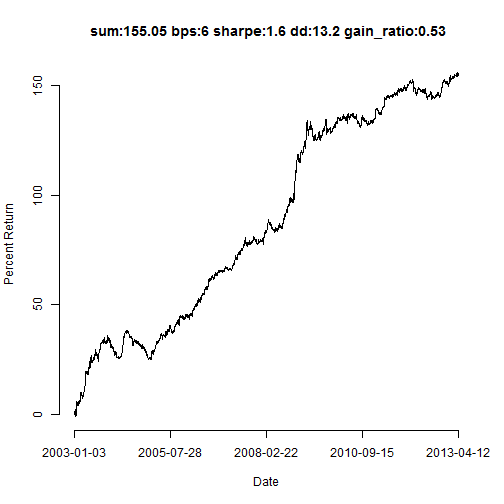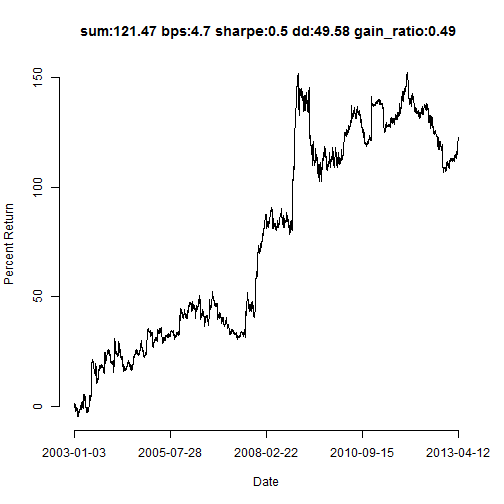These company tickers are XRX and THC, respectively.

One can also explore the portfolio of individual residual strategies when selecting for each one of them whether to mean revert or not, as we did for the individual stocks above. With hindsight this leads to the following returns: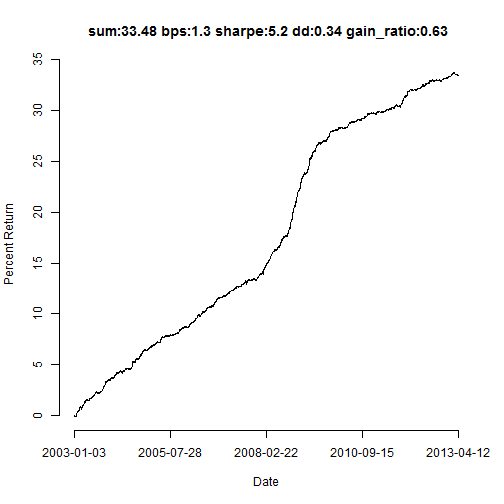But again, choosing between momentum and mean reversion for each redisual portfolio without hindsight is not practical.

The results “with hindsight” may give the impression that, even though one cannot reach those results in practice, there is a lot of potential. Afterall one only has to select 423 binary variables for the entire 10 years of data: whether to follow a mean reversion or a momentum strategy for each individual stock or residual portfolio for the entire 10 years period. At first glance, making only a “423 bits” decision (you can think of it as if you “only see 423 bits of information for the entire 10 years for all 423 stocks, namely for 1093878 real numbers!”) does not seem much at all - especially if this data is “close to random” (note: known risk factors, such as the momentum one, indicate this is not the case - depending on how one models the series). But maybe this is indeed as many bits of information as one could possibly need to “know all about the S&P 500 stocks for 10 years”…

As always, one has to be very aware of the signal to noise ratio in the data one explores. This is what “fooled by randomness” can really mean.

## Some Lessons Learned

• Basic analysis of daily stock returns.

• There appear do be market regimes.

• The “equally weighted market” is the first Principal Component of the daily returns data.

• Example of statistical estimation of, what one could call, “risk factors”.

• Example mean reverting or momentum daily trading strategies.

• It only take a few bits of information with hindsight to get fooled by randomness with this data.